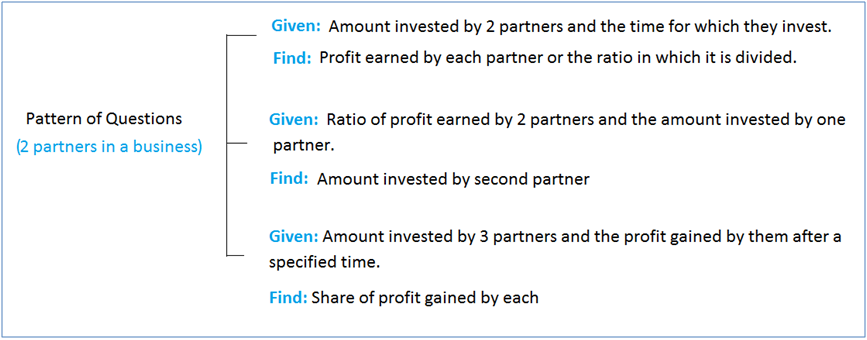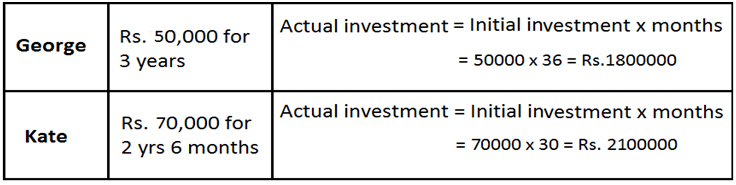# Partnership - Aptitude test, questions, shortcuts, solved example videos

Video on Partnership - shortcuts, tips and tricks

## Partnership

Important terms:

1) Partnership: If two or more than two persons run a business jointly, then they are called partners and the deal is known as partnership. There are two types of partnership: simple partnership and compound partnership.

2) Simple partnership:

a) In this type of partnership, the capitals of each of the partners are invested for same time.
b) Gains or losses are divided among the partners in the ratio of their investments.

3) Compound partnership:

a) In this type of partnership, the periods of investments are unequal.
b) Equivalent capitals for a unit of time are calculated by multiplying the capital with number of units it was in business.

4) Working partner: Partner managing the business

5) Sleeping partner: Partner investing money

Quick tips and tricks:

Ratio of division of gains:

1) Simple partnership: Partners invest for same time.

Suppose P and Q invest Rs. x and Rs. y respectively for a year in a business, at the end of the year:

 P’s share of profit = x Q’s share of profit y

x : y = P’s share of profit : Q’s share of profit

2) Compound partnership: When partners invest for different time periods, then equivalent capitals are calculated for a unit of time by taking (capital x number of units of time).

Suppose P and Q invest Rs. x for a months and Rs. y for b months respectively then,

 P’s share of profit = xa Q’s share of profit yb

Capital (amount) of P x Time period of P: Capital (amount) of Q x Time period of Q = P’s share of profit : Q’s share of profit

When n number of partners invests for different time periods T, then

A1T1: A2T2: A3T3 ……: AnTn = P1: P2: P3....: Pn

A is the amount, T is the time period and P is the profit earned.

Question Variety

Type 1: Simple Partnership:
a) 2 partners join at same time to start a business
b) 3 partners join at same time to start a business

a) Two Partners Join at Same Time to Start a Business:

Note: Different cases/types: The questions are twisted in exams in the following different forms shown below:Q 1. Smith and Kate started a business investing Rs. 84,000 and Rs. 28,000 respectively. In what ratio the profit earned after 2 years be divided between Smith and Kate respectively?

a. 2 : 3
b. 3 : 1
c. 13 : 3
d. None of these
View solution

Correct Option: (b)

Hint:

 P’s share of profit = x - - - - - - (x and y are investments) Q’s share of profit y

x : y = P’s share of profit : Q’s share of profit
Therefore,
 Smith’s share of profit = 84000 = 3 Smita’s share of profit 28000 1
The profit earned after 2 years will be divided between Smith and Kate in the ratio of 3 : 1.

Q 2. Sham and Ram invested in a business and gained a profit which was divided in the ratio of 2 : 3. If Sham invested Rs. 40,000, then what was the amount invested by Ram?

a. Rs. 30,000
b. Rs. 45,000
c. Rs. 60,000
d. Rs. 75,000
View solution

Correct Option: (c)

Hint:

 P’s share of profit = x - - - - - - (x and y are investments) Q’s share of profit y

Let the amount invested by Ram be Rs. X
Therefore,
 Sham’s share of profit = Sham’s investment Ram’s share of profit Ram’s investment
 2 = 40000 3 X
X = Rs. 60,000
Amount invested by Ram = Rs. 60,000

b) Three partners join at same time to start a business

Q 3. John, Tyson and Mike started a business by investing Rs.1,00,000, Rs. 1,50,000 and Rs. 1,75,000 respectively. Find the share of Mike, out of an annual profit of Rs. 46,000.

a. Rs. 15941. 93
b. Rs. 16943. 59
c. Rs. 18941. 17
d. Rs. 19971. 03
View solution

Correct Option: (c)

Here, three partners invest together and earn a profit of Rs. 46,000 at the end.
Hint: Ratio of shares of John, Tyson and Mike = Ratio of their investments
Therefore,
100000 : 150000 : 175000 = 4 : 6 : 7
Now, we have to calculate share belonging to each person from all the shares considering the annual profit.
Total shares = 4 + 6 + 7 = 17 shares

 John’s Share = 4 × 46000 = Rs. 10823.53 17
 Tyson’s Share = 6 × 46000 = Rs. 16235.294 17
 Mike’s Share = 7 × 46000 = Rs. 18941.176 17

Q 4. Harry, John and Smith start a shop by investing Rs. 27,000. Rs. 72,000 and Rs. 81,000 respectively. At the end of the year, the profit was distributed among them and Smith earns the share of Rs. 36,000. Find the total profit.

a. Rs. 1,10,000
b. Rs. 1,2,5000
c. Rs. 98,000
d. Rs. 80,000
View solution

Correct Option: (d)

Hint: Ratio of shares of Harry, John and Smith = Ratio of their investments
Harry : John : Smith = 27000 : 72000 : 81000 = 3 : 8 : 9

Given: Share of profit earned by Smith = Rs. 36,000
Total no. of shares = 3 + 8 + 9 = 20 shares

 Smith’s share = 9 20
Let total profit = Rs. X
 36000 = 9 X 20
 X = 36000 × 20 = 80000 9
Total profit = Rs. 80,000

Type 2: Compound Partnership:
a) 2 partners join at different time in a business
b) 2 partners join at same time and 3rd partners joins the same business later
c) 3 Partners join at different time

A) 2 Partners Join at Different Time in a Business
1) Given: Investment of partners and time of each joining partner. Total profit earned after specified time.
Find: Share of profit
2) Given: Investment of two partners and time of joining partner. Ratio of profit earned.
Find: Partner 2nd joining the business with 1st partner.

Q 5. George started a hardware business by investing Rs. 50,000. After six months, Kate joined him with a capital of Rs. 70,000. After 3 years, they earned a profit of Rs. 25,000. Find George’s share in profit.

a. Rs. 13000.00
b. Rs. 13224.65
c. Rs. 12500.50
d. Rs. 11538.46
View solution

Correct Option: (d)

This type of partnership is called as compound partnership. George invested for 3 years (36 months) and Kate for 2 years 6 months (30 months). After George’s investment for 36 months and Kate’s investment for 30 months, both get a profit of Rs. 25,000.

Hint:

 George’s share of profit = Initial investment of George × Time Kate’s share of profit Initial investment of Kate × Time

Always try to simplify this type of numerical by arranging them in table format as shown below:Actual investment ratio of George and Kate = Actual investment of George = 1800000 = 6 Actual investment of Kate 2100000 7
 George’s share of profit = 6 × 25000 = Rs. 11538.46 13

Q 6. Rohan began a business with Rs. 85,000 and was joined by Sanket with Rs. 42,500. If the profits at the end of the year are divided in the ratio of 3 : 1, then find for how much period of time did Sanket join Rohan?

a. 10 months
b. 9 months
c. 8 months
d. 7 months
View solution

Correct Option: (c)

Hint: This is a type of compound partnership. Hence,

 P’s share of profit = xa - - - - - - (x and y are investments and a, b are time) Q’s share of profit yb

Let us assume the joining period of Sanket = X
Given:
1) Profits at the end of the year are divided in the ratio of 3 : 1
2) Rohan’s earns a profit after 12 years

Therefore,
 Rohan’s share of profit = xa Sanket’s share of profit yb
 3 = 85000 × 12 1 42500 × X
X=8
Sanket joined for 8 months.

b) 2 Partners Join at Same Time and 3rd Partners Joins the Same Business Later

Q 7. Two partners X and Y started a business by investing in the ratio of 5 : 8. Z joined them after 8 months investing an amount equal to that of Y. At the end of the year, 20 % profit was earned which is equal to Rs. 98,000. Find the amount invested by Z.

a. Rs. 213818.16
b. Rs. 223878.12
c. Rs. 203818.16
d. Rs. 219818.13
View solution

Correct Option: (a)

Let the total profit be p.
Given: 20 % profit is equal to Rs. 98,000
20 % of p = 98000
p = 490000
Capital of X = 5 x
Capital of Y = 8 x
Capital of Z = 8 x
Therefore,
(5x × 12) + (8x × 12) + (8x + 8) = 490000 × 12
220x = 5880000
x = Rs. 26727.27
We have find the amount invested by z.
8x = 8 x 26727.27 = Rs. 213818.16

C) 3 Partners Join at Different Time

Q 8. Three partners partnered for 14 months, 8 months and 7 months respectively. Find the ratio of their investments, if they shared a profit in the ratio 5 : 7 : 8.

a. 11 : 19 : 37
b. 17 : 25 : 57
c. 15 : 33 : 68
d. 20 : 35 : 64
View solution

Correct Option: (d)

Hint: Capital (amount) of P x Time period of P: Capital (amount) of Q x Time period of Q = P’s share of profit : Q’s share of profit
Let us assume the investments of 3 partners as x, y and z.
Therefore,
14x : 8y : 7z = 5 : 7 : 8

 14x = 5 8y 7
70x=40y-----(1)
 14x = 5 7z 8
112x=35z----(2)
From (1) and (2), we get
 y = 7 x and z = 16 x 4 5

We have to find the ratio of investments of 3 partners.
 x : y : z = x : 7 x : 16 x 4 5
Solving we get, the solution as:
x : y : z = 20 : 35 : 64

Logarithm - Aptitude test, questions, shortcuts, solved example videos
This is the aptitude section on Logarithm with tips, tricks and questions and explanations for various interview, competitive examination and entrance test.